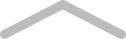# How To Talk To A Police Radio# Chapter 1 - Number Plates

A = Alpha

B = Bravo

C = Charlie

D = Delta

E = Echo

F = Foxtrot

G = Golf

H = Hotel

I = Indian

J = Juliet

K = Kilo

L = Lima

M = Mike

N = November

O = Oscar

P = Papa

Q = Quebec

R = Romeo

S = Sierra

T = Tango

U = Uniform

V = Victor

W = Whiskey

X = X-ray

Y = Yankee

Z = ZuluLog in or Join Tablo to comment on this chapter...
~
69 mins to go